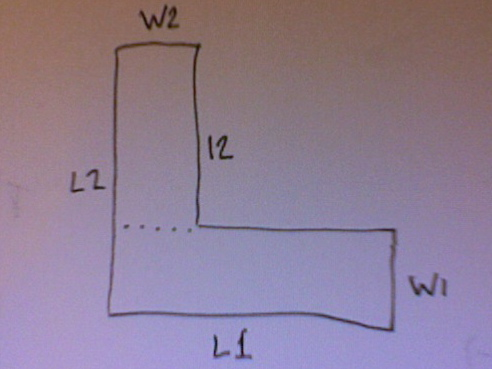# How To Find The Area Of A L-shaped Room?

Measure the long walls of the L. Call those L1, L2.
Measure the width of each leg. Call those W1, W2, where W1 is the width of the leg L1, and W2 is the width of the leg L2.

The area of the L shape will be either of ...

area = L1*L2 - (L1-W2)(L2-W1)

area = L1*W1 + L2*W2 - W1*W2

If you compute it both ways and get different answers, your math is off.
_____
Alternatively, measure the long wall of one leg (L1), and the width of that leg (W1). (The area of that would be L1*W1.) Then measure the short wall of the other leg (l2) and the width of that leg (W2). The area of that would be l2*W2. The total area is the sum of those products,
area = L1*W1 + l2*W2.

Perhaps the picture will help.thanked the writer.
renee cardwell commented
The area is the length * width
thanked the writer.